# 2021年蓝桥杯第十届软件类省赛 c++A组 第一场（口胡题解） 未完成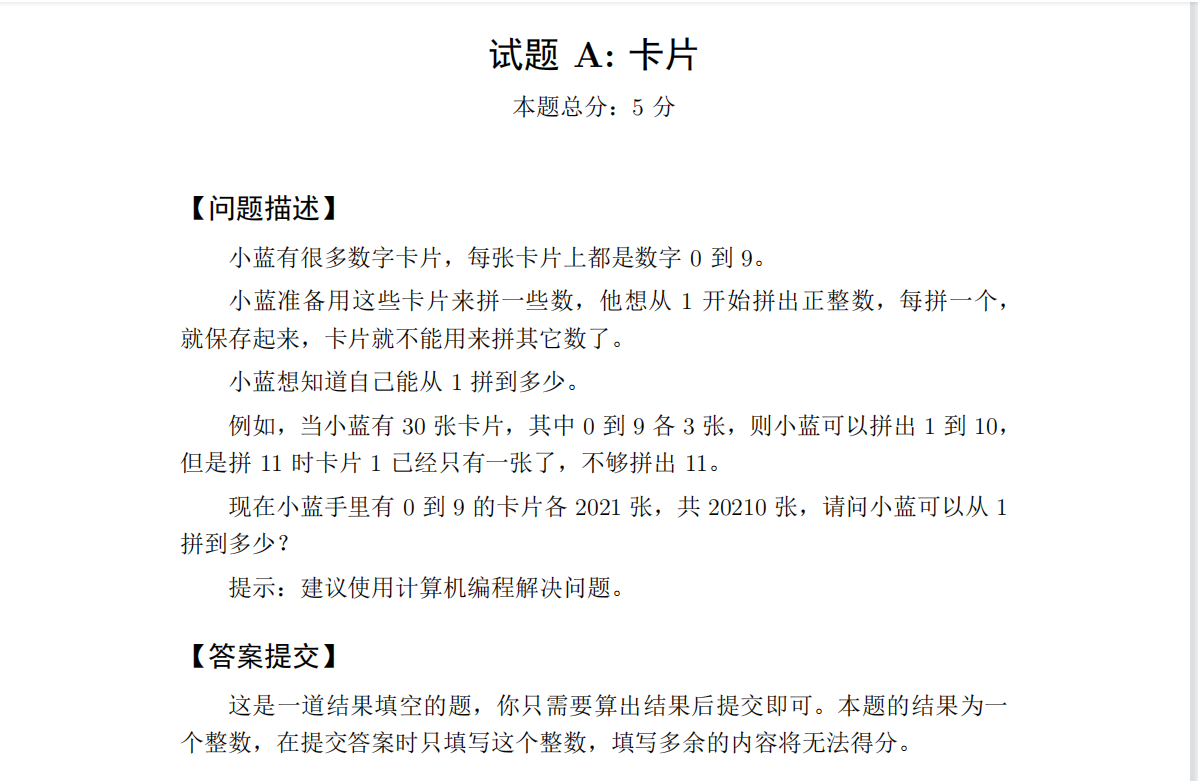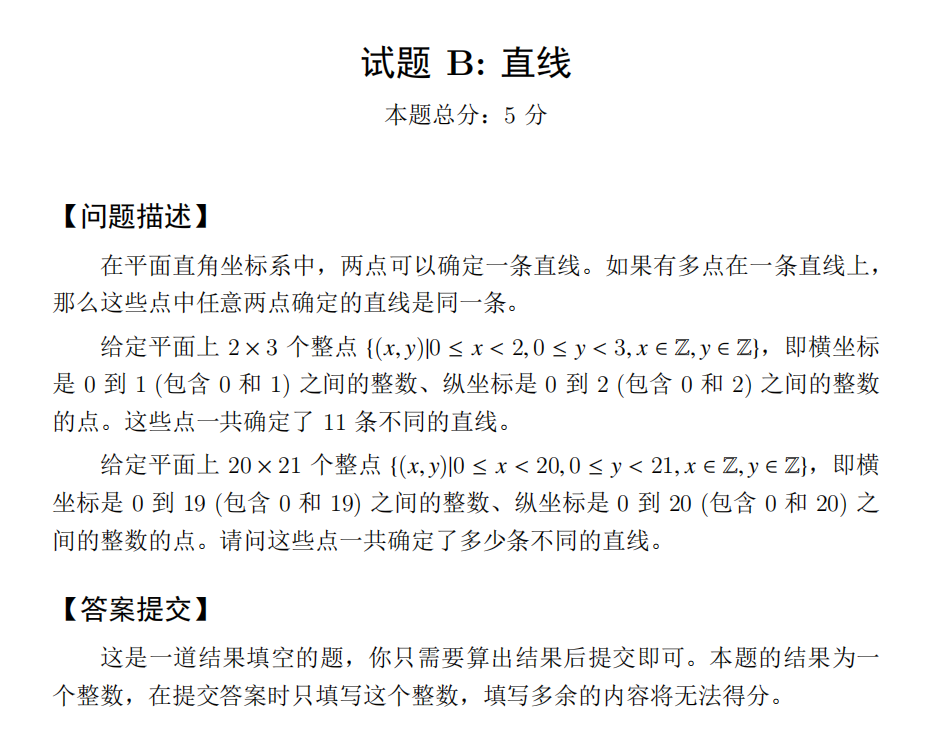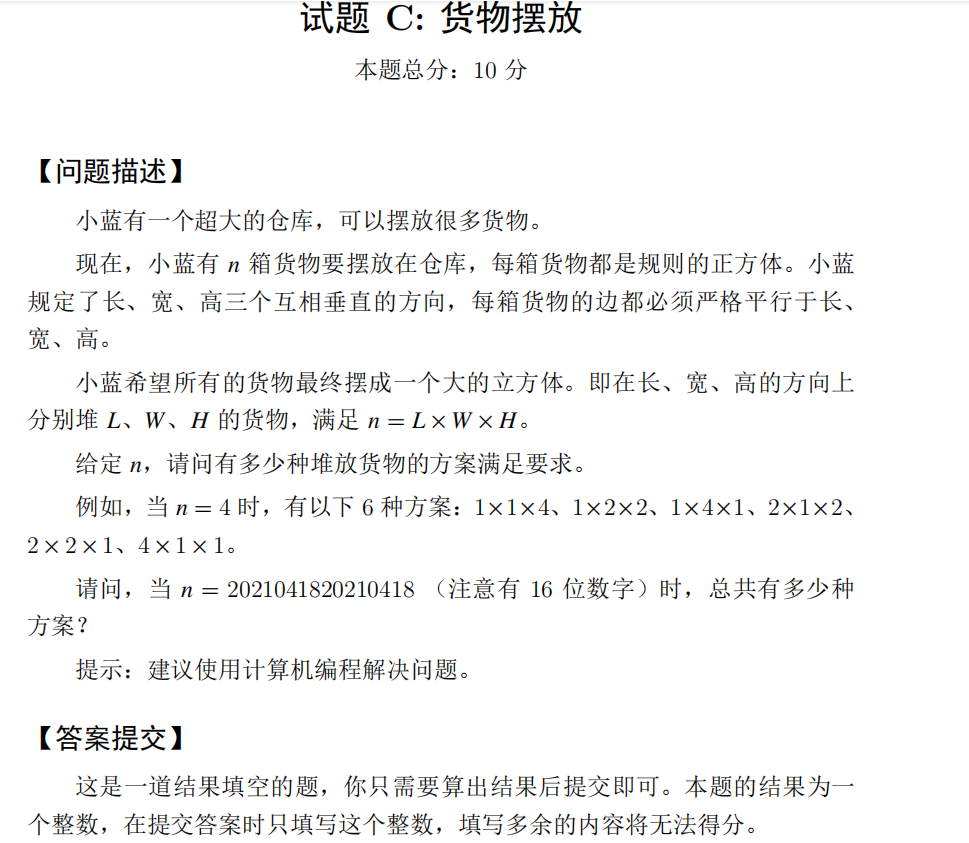$n$的质因数个数最多就$logn$个，所以这里直接暴力找找就好

1，直接对于所有质因数统计，再对相同质因数去重

$ans = \frac{\binom{[\sum t(i)] - 1}{2}}{\prod{t(i)!}}$

$t(i)$表示第$i$种质因数的个数

2,对不同质因数分开统计

$ans = \prod{\binom{t(i) - 1}{2}}$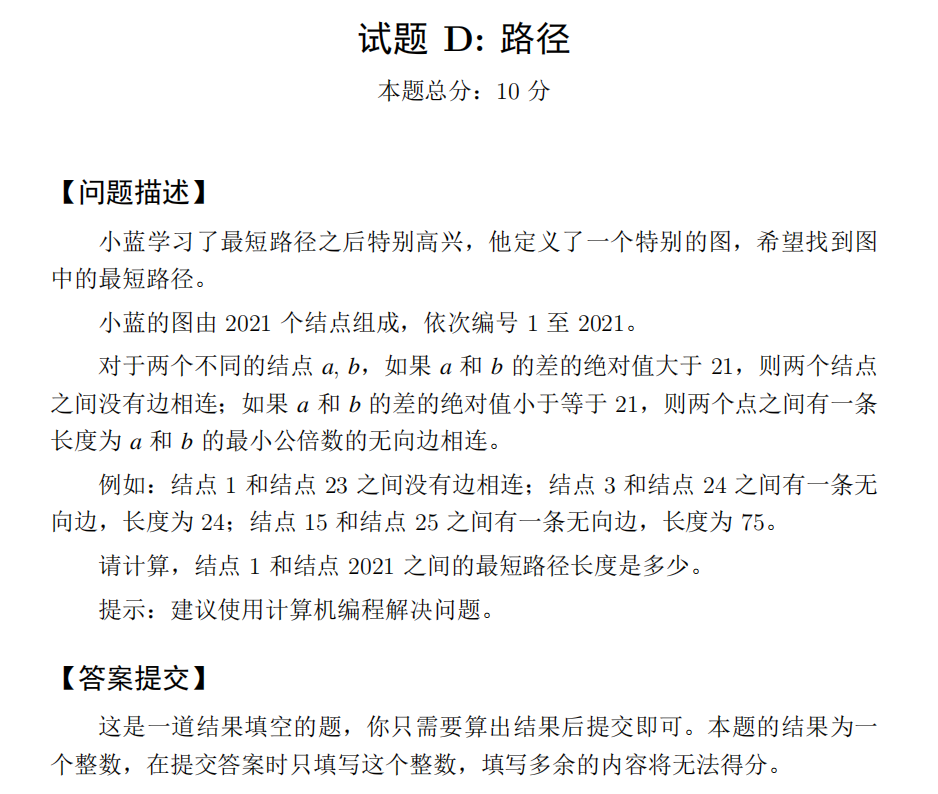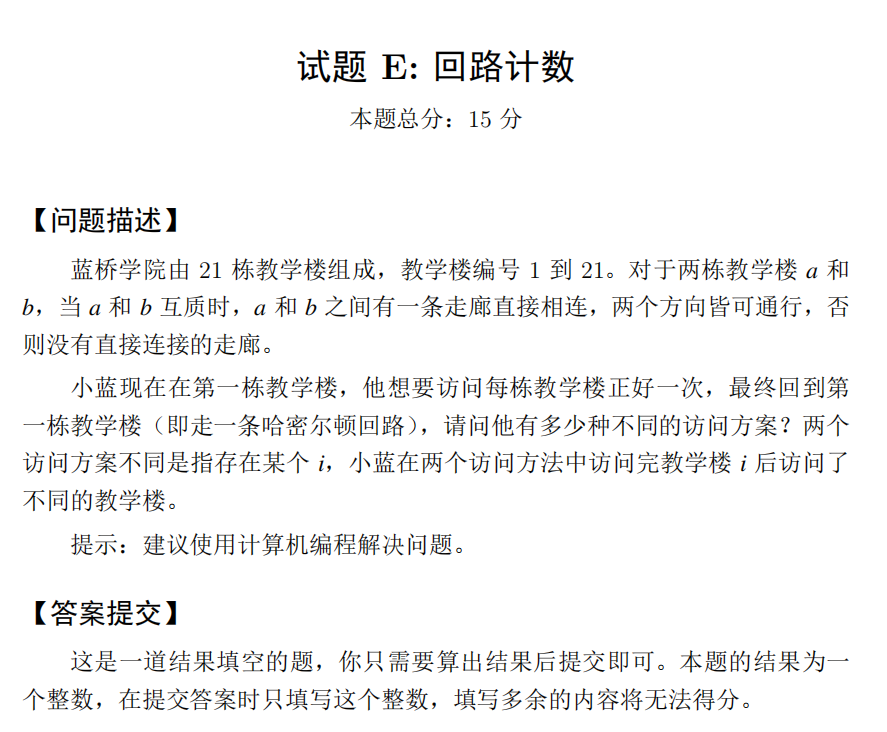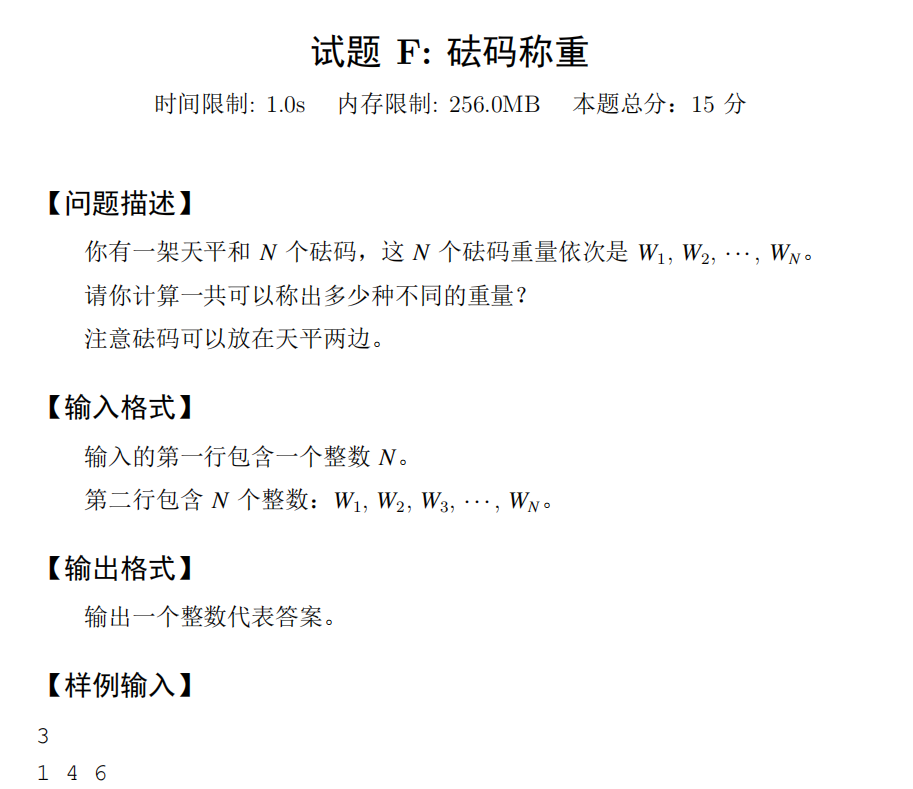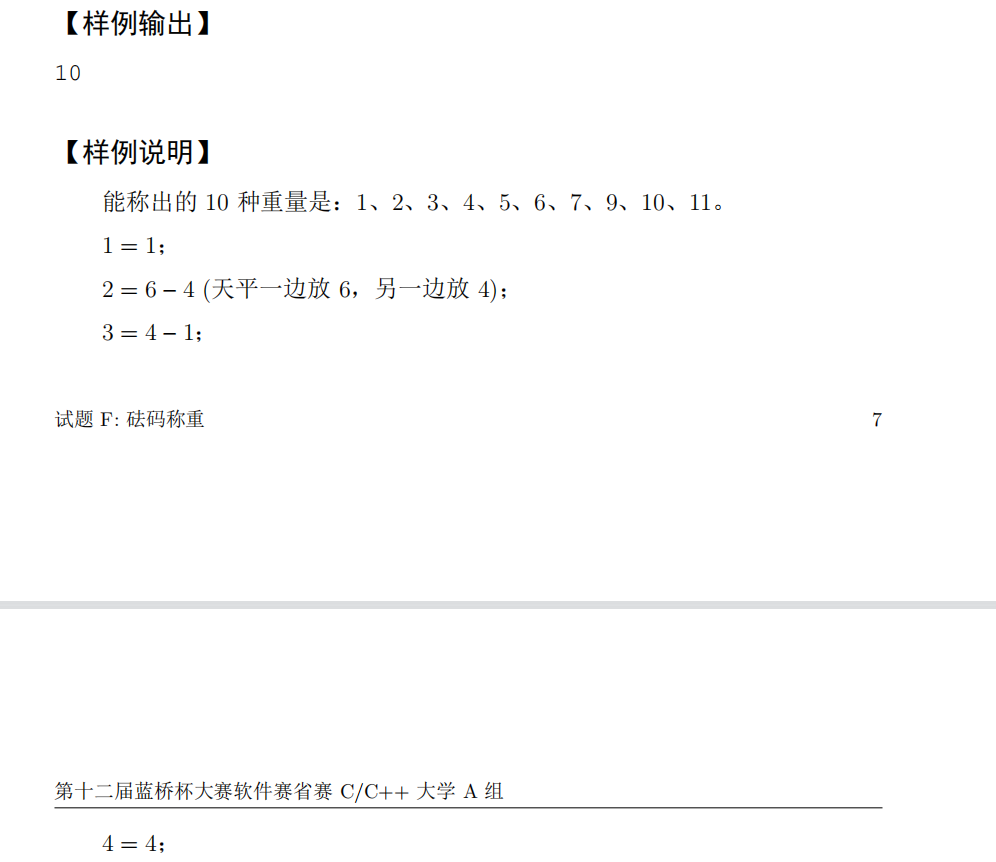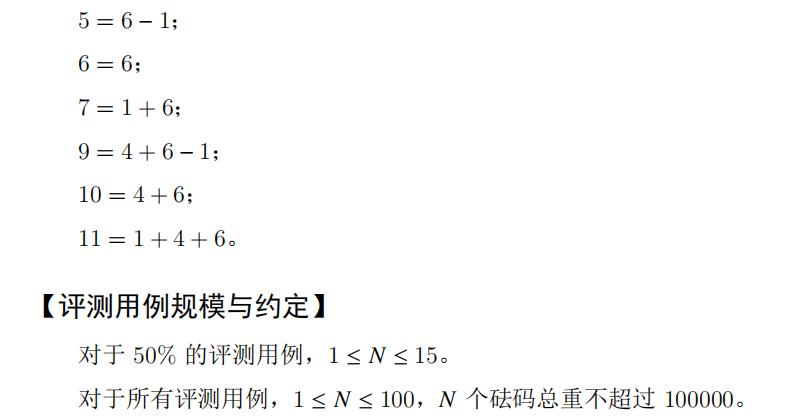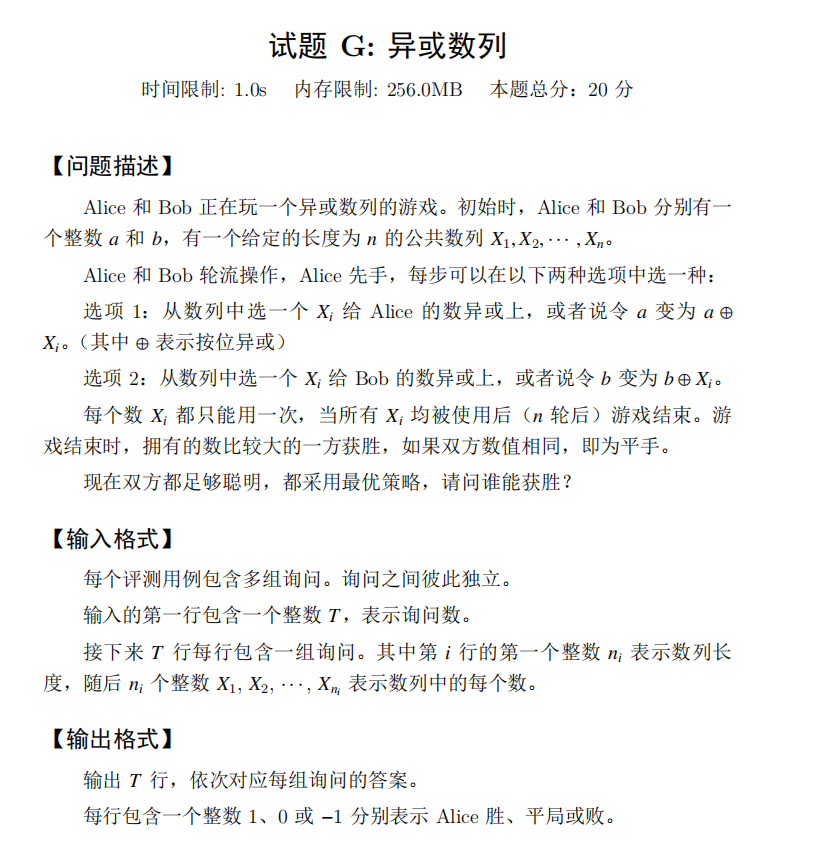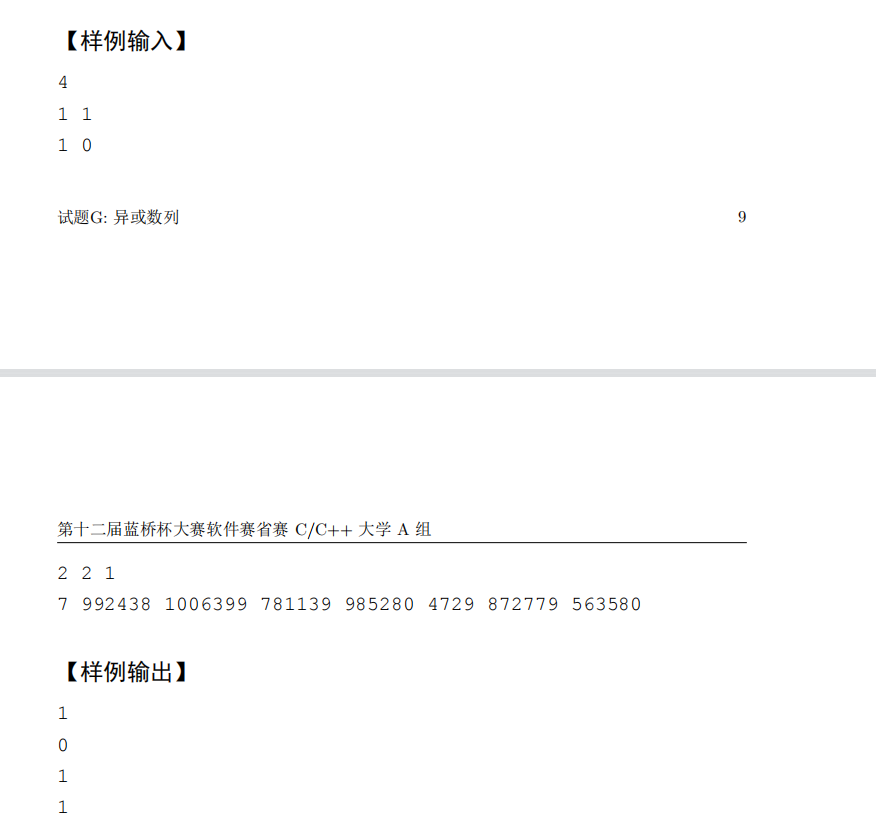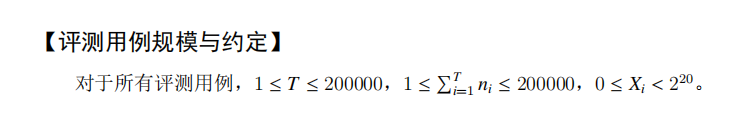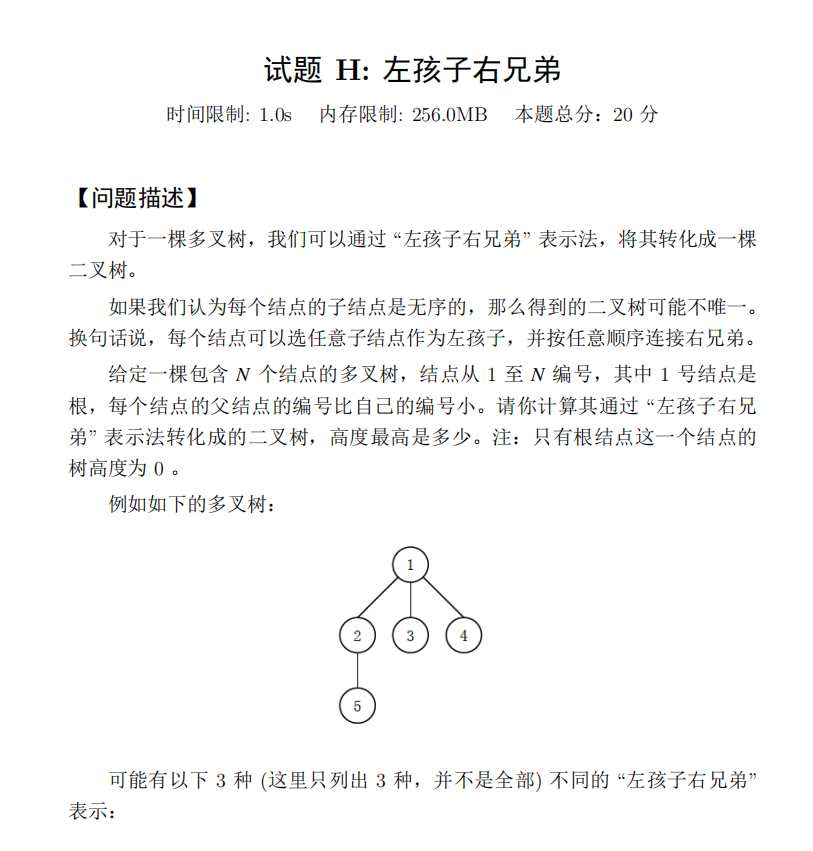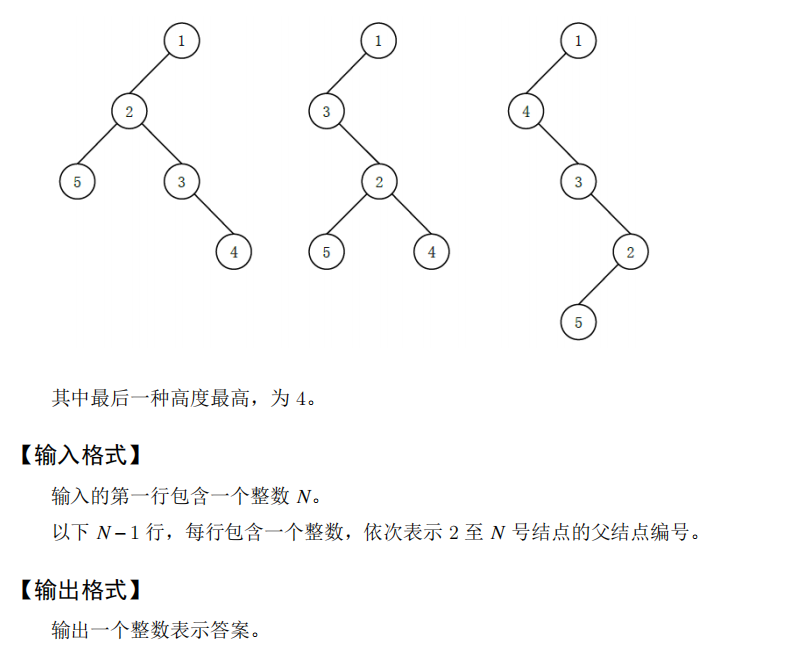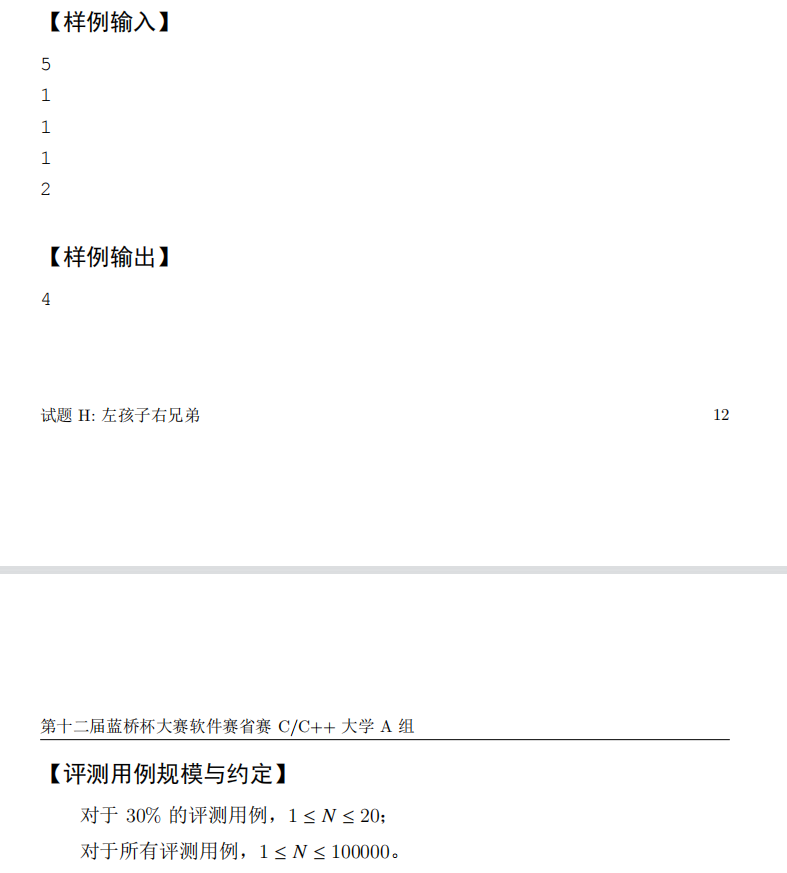$f[i]$表示以$i$为根的子树重新组合后的最大高度。那么考虑一个贪心的做法。
$f[i]$从小到大排序，把高的子树接在矮的子树下面。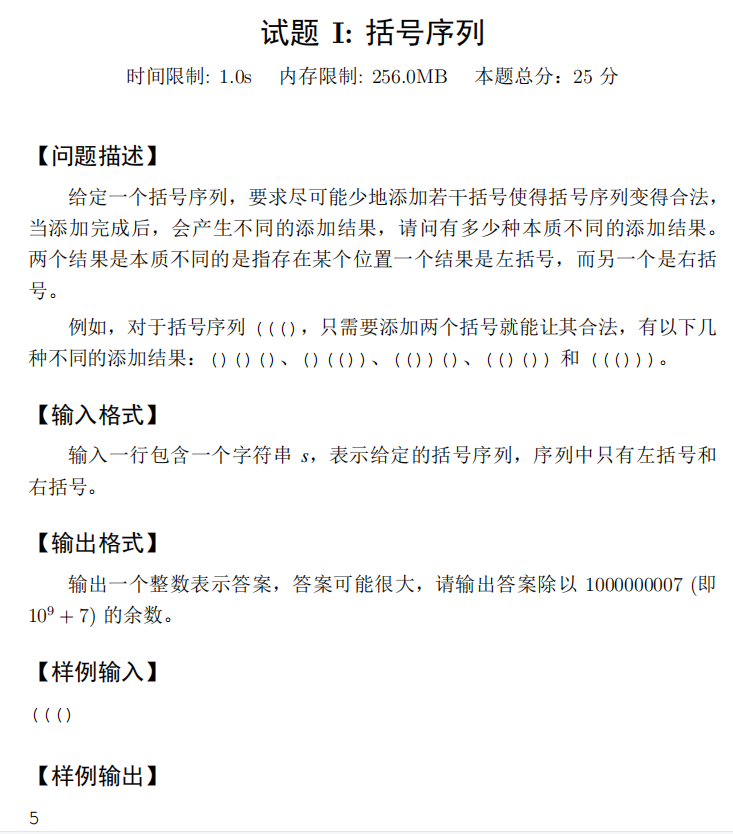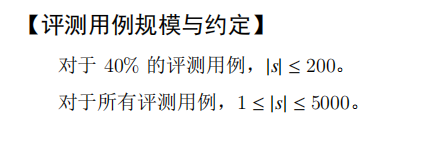$f[i][j] = \sum_{0 <= k <= min(lim[i - 1], j)} f[i - 1][k]$

i这一维可以滚掉，因为第二维是大的数用到小的数，所以从后向前枚举。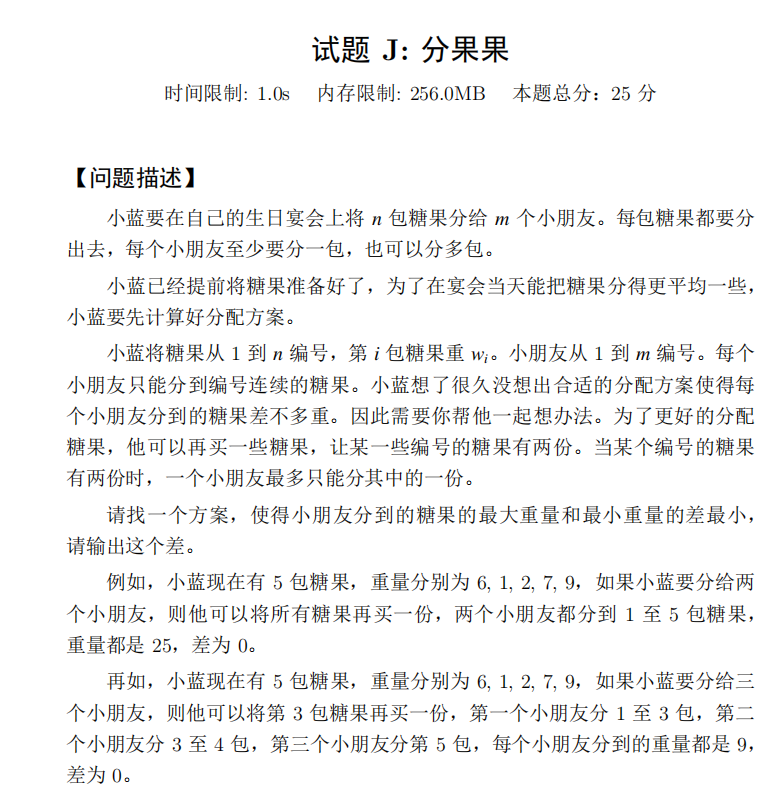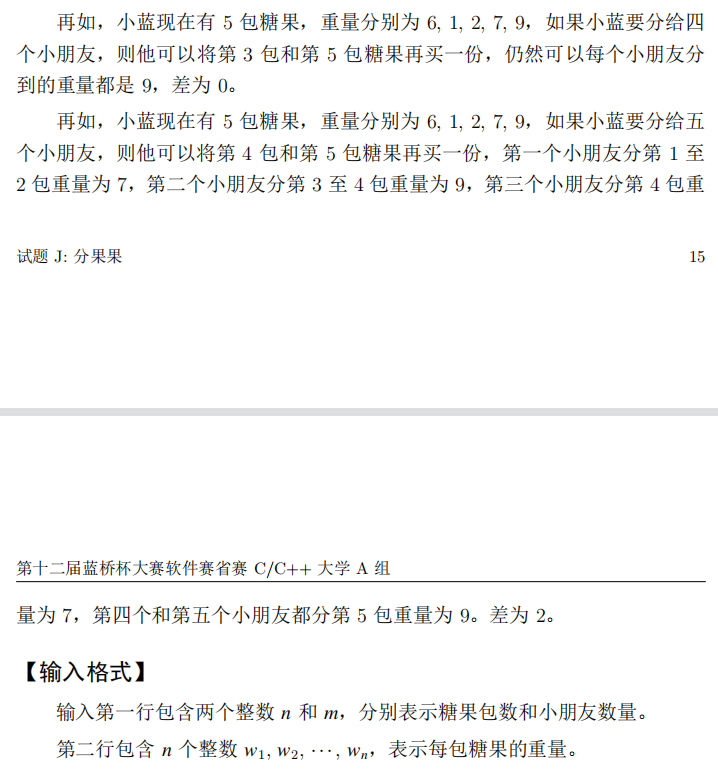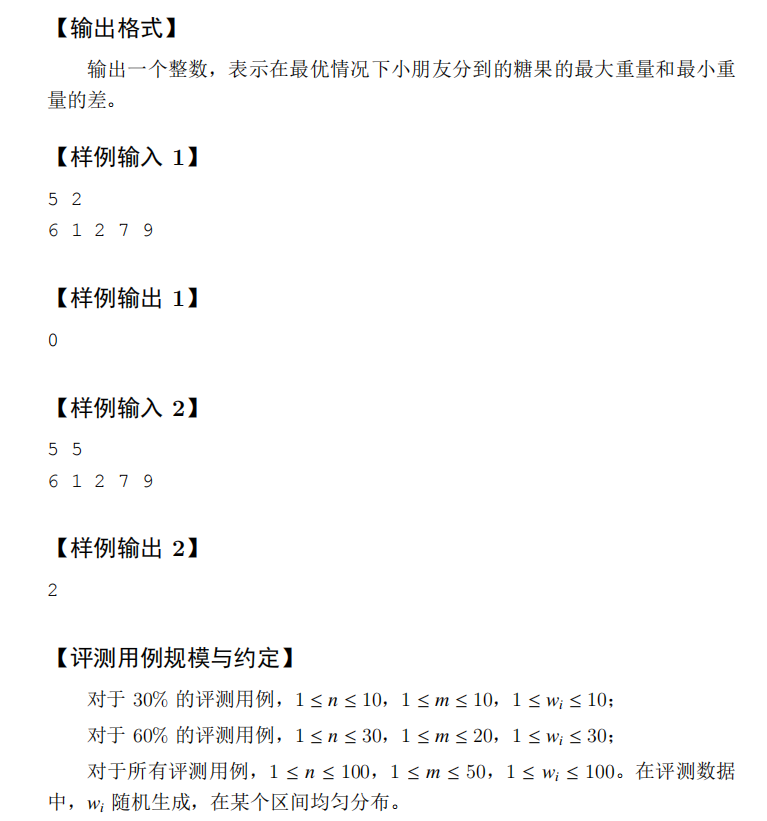posted @ 2021-05-08 23:00  ww3113306  阅读(422)  评论(2编辑  收藏  举报